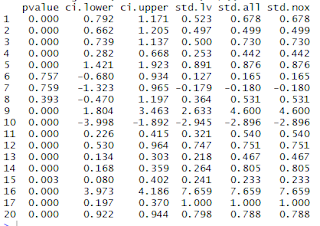# How to get a confidence interval around the standardized estimates?

233 views

### Amonet

Jul 27, 2018, 11:12:14 AM7/27/18
to lavaan
Hi,

I calculated the Omega2 coefficient using estimates from my CFA. The syntax is as follows:

Model1 <- 'LV =~ A1*Item1 + C1*Item3 + D1*Item4 + E1*Item5 + F1*Item6

Item1 ~ a1*1
Item3 ~ c1*1
Item4 ~ d1*1
Item5 ~ e1*1
Item6 ~ f1*1

A1 + C1 + D1 + E1 + F1 == 5

Item1 ~~ ME1*V_0_1
Item3 ~~ ME3*V_0_3
Item4 ~~ ME4*V_0_4
Item5 ~~ ME5*V_0_5
Item6 ~~ ME6*V_0_6

a1 + c1 + d1 + e1 + f1 == 0

LV ~ 1

LV ~~ LV

Omega := (A1+C1+D1+E1+F1)^2 / ((A1+C1+D1+E1+F1)^2+(ME1+ME3+ME4+ME5+ME6))
'
fit1 <- fit1 <- lavaan(model = Model1, data = data, estimator = 'MLR')

The intercepts are estimated of each item, albeit that it's not really needed in this model. Just included them for completeness.
As you can see, I used effects coding.

Now, what I am wondering about: is it possible to get the confidence interval of a standardized estimate in Lavaan?
Using: parameterestimates(fit1, standardized = TRUE), I get the following estimates (I omitted some of the columns).The CI.lower and CI.upper are for the unstandardized estimate. I am particularly interested in the last row (20). This is omega that is defined in the model syntax. The 0.798 (std.lv) is the standardized estimate I would like the CI for. I noticed that using semTools::reliability(fit1) gives me a similar standardized estimate for Omega2, and so does the estimate from the MBESS package.

Using the MBESS package, I can also get the confidence interval of Omega2, which uses bootstrapping to produce the CI (although it can be set to compute differently also). The 95% CI I get there: 0.737 to 0.860. The 95% CI of the unstandardized estimate that I computed using Lavaan, gave me the wrong impression. I thought it was a rather high reliability with a small interval, but the standardised estimate is clearly wider and also smaller.

Thanks,
Amonet

### Mauricio Garnier-Villarreal

Jul 27, 2018, 1:58:15 PM7/27/18
to lavaan

standardizedsolution(fit1, level=.95)